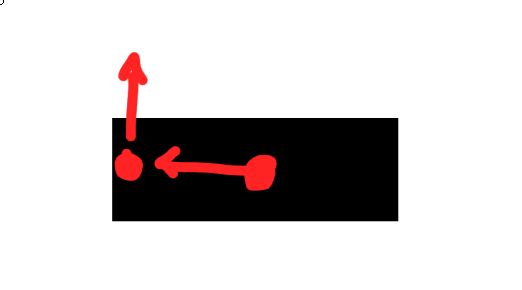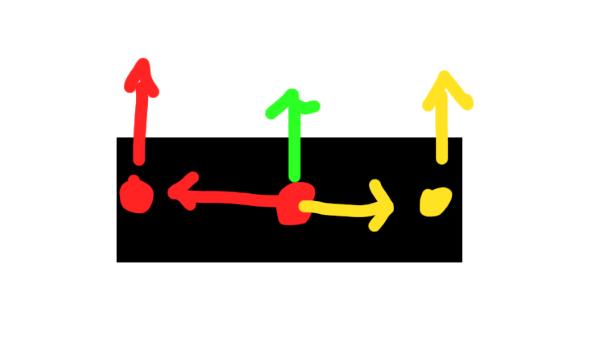# Is there any way to detect when anchored part is touching?

hey guys, so i have a little bug

so, im using an raycast. But the ray cast only rays on the center of the part, not on both sides. Is There any way to solve this, thx!

Can you explain more? You want to raycast it from the sides?

i want, a solution that makes the center part cant move when detects wall.

Thing is you didn’t even tell us how your part moves

here ima share the script

so basically the part moves with uis ( userinputservice ), and move with cframe

``````local char = workspace:FindFirstChild('char')
local uis = game:GetService('UserInputService')
local run = game:GetService('RunService')

local keys = {'W','S','A','D'}
local ckeys = {}
local cantw = {}

local wspeed = .2

uis.InputBegan:Connect(function(k,g)
if table.find(keys,k.KeyCode.Name) then
if table.find(keys,k.KeyCode.Name) == 1 and not table.find(ckeys,k.KeyCode.Name) then
table.insert(ckeys,k.KeyCode.Name)
end
if table.find(keys,k.KeyCode.Name) == 2 and not table.find(ckeys,k.KeyCode.Name) then
table.insert(ckeys,k.KeyCode.Name)
end
if table.find(keys,k.KeyCode.Name) == 3 and not table.find(ckeys,k.KeyCode.Name) then
table.insert(ckeys,k.KeyCode.Name)
end
if table.find(keys,k.KeyCode.Name) == 4 and not table.find(ckeys,k.KeyCode.Name) then
table.insert(ckeys,k.KeyCode.Name)
end
end
end)

uis.InputEnded:Connect(function(k,g)
if table.find(keys,k.KeyCode.Name) then
if table.find(keys,k.KeyCode.Name) == 1 and table.find(ckeys,k.KeyCode.Name) then
table.remove(ckeys,table.find(ckeys,k.KeyCode.Name))
end
if table.find(keys,k.KeyCode.Name) == 2 and table.find(ckeys,k.KeyCode.Name) then
table.remove(ckeys,table.find(ckeys,k.KeyCode.Name))
end
if table.find(keys,k.KeyCode.Name) == 3 and table.find(ckeys,k.KeyCode.Name) then
table.remove(ckeys,table.find(ckeys,k.KeyCode.Name))
end
if table.find(keys,k.KeyCode.Name) == 4 and table.find(ckeys,k.KeyCode.Name) then
table.remove(ckeys,table.find(ckeys,k.KeyCode.Name))
end
end
end)

run.Heartbeat:Connect(function()
if table.find(ckeys,'W') and not table.find(cantw,'w') then
char.CFrame *= CFrame.new(0,0,-wspeed)
char.Orientation = Vector3.new(0,0,0)
game:GetService('TweenService'):Create(workspace.CurrentCamera,TweenInfo.new(.3),{CFrame = char.CFrame * CFrame.Angles(math.rad(-90),0,0) * CFrame.new(0,0,16)}):Play()
wait()
workspace.CurrentCamera.CameraType = Enum.CameraType.Scriptable

end
if table.find(ckeys,'S') and not table.find(cantw,'s') then
char.CFrame *= CFrame.new(0,0,wspeed)
char.Orientation = Vector3.new(0,0,0)
game:GetService('TweenService'):Create(workspace.CurrentCamera,TweenInfo.new(.3),{CFrame = char.CFrame * CFrame.Angles(math.rad(-90),0,0) * CFrame.new(0,0,16)}):Play()
wait()
workspace.CurrentCamera.CameraType = Enum.CameraType.Scriptable

end
if table.find(ckeys,'D') and not table.find(cantw,'d') then
char.CFrame *= CFrame.new(wspeed,0,0)
char.Orientation = Vector3.new(0,0,0)
game:GetService('TweenService'):Create(workspace.CurrentCamera,TweenInfo.new(.3),{CFrame = char.CFrame * CFrame.Angles(math.rad(-90),0,0) * CFrame.new(0,0,16)}):Play()
wait()
workspace.CurrentCamera.CameraType = Enum.CameraType.Scriptable

end
if table.find(ckeys,'A') and not table.find(cantw,'a') then
char.CFrame *= CFrame.new(-wspeed,0,0)
char.Orientation = Vector3.new(0,0,0)
game:GetService('TweenService'):Create(workspace.CurrentCamera,TweenInfo.new(.3),{CFrame = char.CFrame * CFrame.Angles(math.rad(-90),0,0) * CFrame.new(0,0,16)}):Play()
wait()
workspace.CurrentCamera.CameraType = Enum.CameraType.Scriptable

end
end)
``````

context:
cantw = cant walk
ckeys = current keys
wspeed = walk speed

the char is that part

the ray script

``````run.Heartbeat:Connect(function()
--print(char:GetTouchingParts())
--if char:GetTouchingParts() ~= nil then
--char.CFrame = workspace.tes2.CFrame
--end
local ry_front = Ray.new(workspace.front.Position,Vector3.new(0,0,-.4))
local ry_back = Ray.new(workspace.back.Position,Vector3.new(0,0,.4))
local ry_right = Ray.new(workspace.right.Position,Vector3.new(.4,0,0))
local ry_left = Ray.new(workspace.left.Position,Vector3.new(-.4,0,0))

local h_front = workspace:FindPartOnRayWithIgnoreList(ry_front,{workspace.front,workspace.char})
local h_back = workspace:FindPartOnRayWithIgnoreList(ry_back,{workspace.back,workspace.char})
local h_right = workspace:FindPartOnRayWithIgnoreList(ry_right,{workspace.right,workspace.char})
local h_left = workspace:FindPartOnRayWithIgnoreList(ry_left,{workspace.left,workspace.char})

if h_front and not table.find(cantw,'w') then
table.insert(cantw,'w')
elseif not h_front and table.find(cantw,'w') then
table.remove(cantw,table.find(cantw,'w'))
end

if h_back and not table.find(cantw,'s') then
table.insert(cantw,'s')
elseif not h_back and table.find(cantw,'s') then
table.remove(cantw,table.find(cantw,'s'))
end

if h_right and not table.find(cantw,'d') then
table.insert(cantw,'d')
elseif not h_right and table.find(cantw,'d') then
table.remove(cantw,table.find(cantw,'d'))
end

if h_left and not table.find(cantw,'a') then
table.insert(cantw,'a')
elseif not h_left and table.find(cantw,'a') then
table.remove(cantw,table.find(cantw,'a'))
end

print(table.concat(cantw,', '))
end)

``````
1 Like

the problem is raycast cant ray on sides, it can only ray on the center of the part

Raycast from the center of the part to each side and check the length of each ray, if the length is too short then that means the part is very close to the wall and disable moving that way

or if you have another way to fix this?, to prevent the part to collide or event “wallglitch”

Ray from the center of the part, set the length to half the part size + some additional length

Or you can raycast from the sides if you wish, but the center is the common point for alldo you mean like this ?

No, make 4 raycasts from the center

All of them start at the center but end in front, back, left, right

You can also make diagonal raycasts if you wish and more, but first let’s fix the issuelike this?

raycast:
red:1
green: 2
yellow: 3 ?

But i Have Another complicated solution

A very quick and easy example is to set each raycast length to be the part’s size / 2, this way the raycast will travel till the face of each side and then add some additional length for the wall check

Still no, 4 raycasts, all of them start in the center but one finishes in the front, one in the back, one in the left and one in the right

Yeah imo you shouldn’t even be using the directional parts for the origin of the Ray, you could just use the center of the block and cast out by a fixed amount like this.

``````local directions = {
Vector3.FromNormalId(Enum.NormalId.Front);
Vector3.FromNormalId(Enum.NormalId.Back);
Vector3.FromNormalId(Enum.NormalId.Left);
Vector3.FromNormalId(Enum.NormalId.Right);
}

COLLISION_BUFFER = 1

local function checkEdges(part, check_magnitude)
local params = RaycastParams.new(); params.FilterType = Enum.RaycastFilterType.Blacklist
params.FilterDescendantsInstances = {part,workspace.char}

local p1 = part.Position

for _, dir in pairs(directions) do
local results = workspace:Raycast(p1, dir*check_magnitude, params)
if (results ~= nil) and (results.Instance ~= nil) then
return false -- did not pass collision check
end
end

return true -- passed collision check
end
``````

Just call
checkEdges(workspace.char.PrimaryPart, workspace.char.PrimaryPart.Size.X / 2 + COLLISION_BUFFER) when you want to see if it’s colliding

2 Likesthis is my solution, seems complicated but yea

That’s not complicated but rather not performance wise, because you can make something easier without that much raycasts

1 Like

thx for the code, ima try to implement this